# Basic Charts¶

The Basic charts build visualizations based on the following types of columns:

• A required binning column, whose values are broken down into discrete values, or bins.

• A required summary column, whose values are aggregated for each bin. Some basics charts allow multiple summary columns.

• An optional grouping column to produce subgroups of bins.

## Chart Layouts¶

### Bar¶

• The Histogram layout puts the binning column on the X axis, the summary column on the Y axis, and creates separate bars for each subgroup for each bin.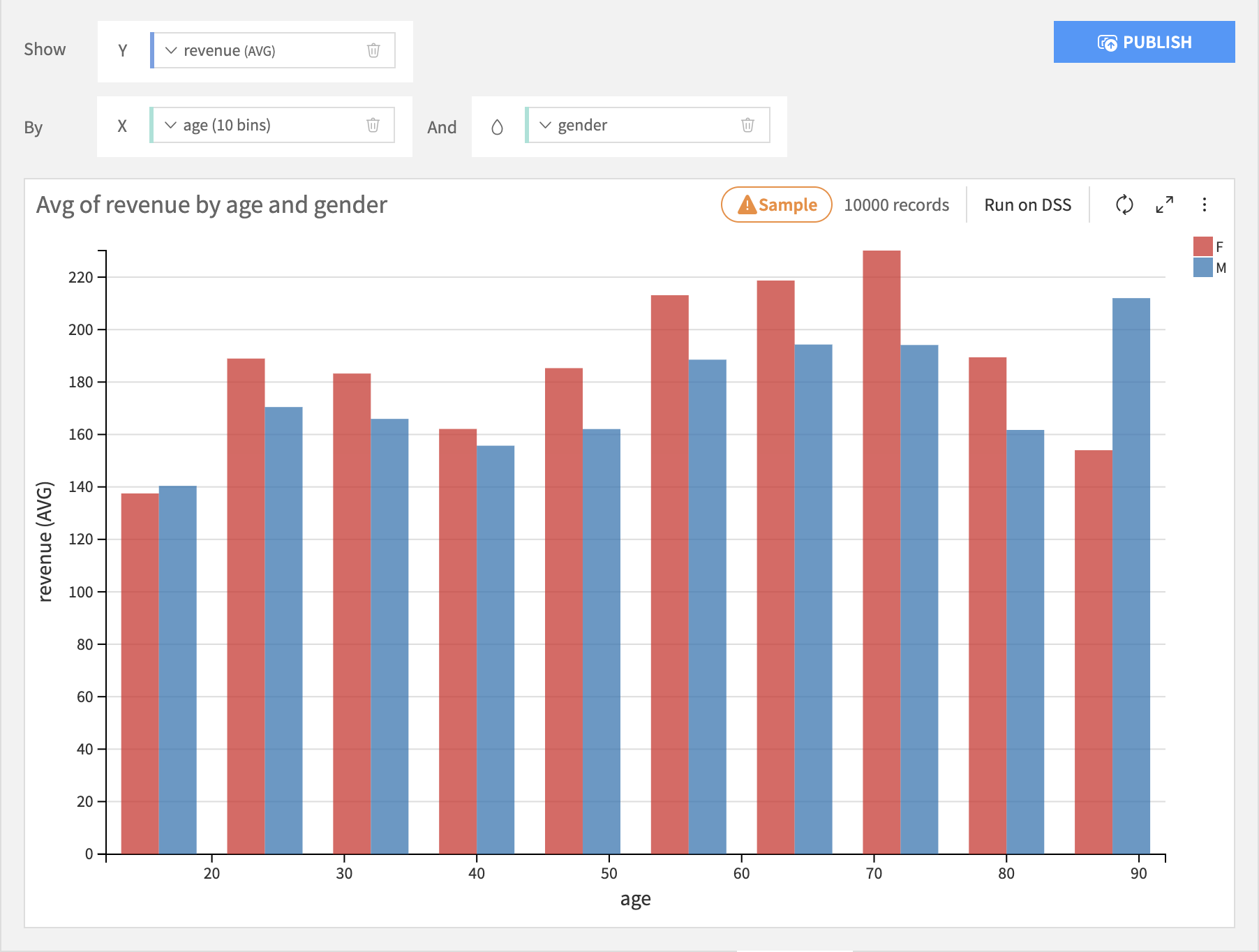• The Stacked layout puts the binning column on the X axis, the summary column on the Y axis, and stacks each subgroup within each bin.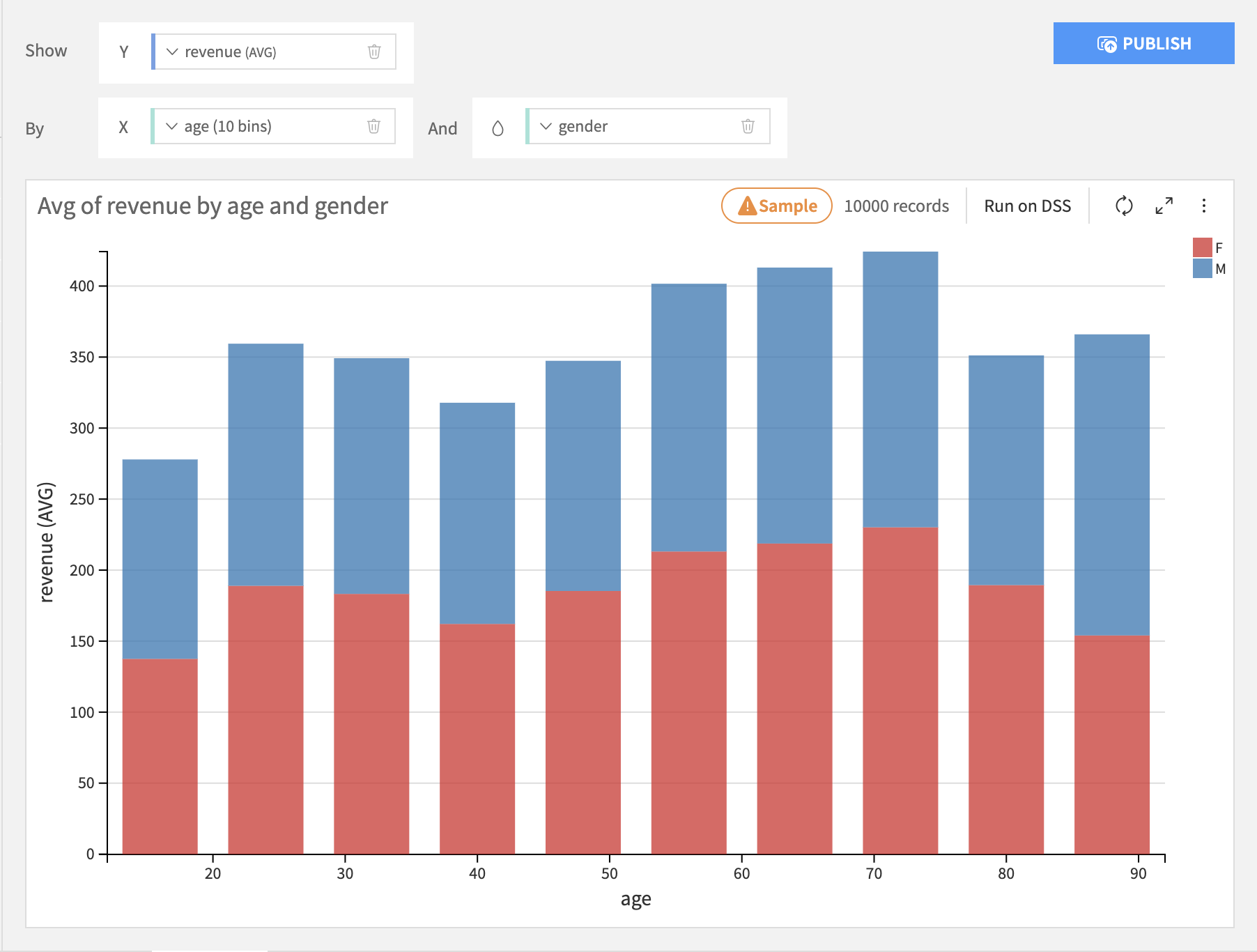• The Stacked 100% is just like the Stacked layout, but the height of each bar is normalized to 100%. This is useful for seeing differences across bins.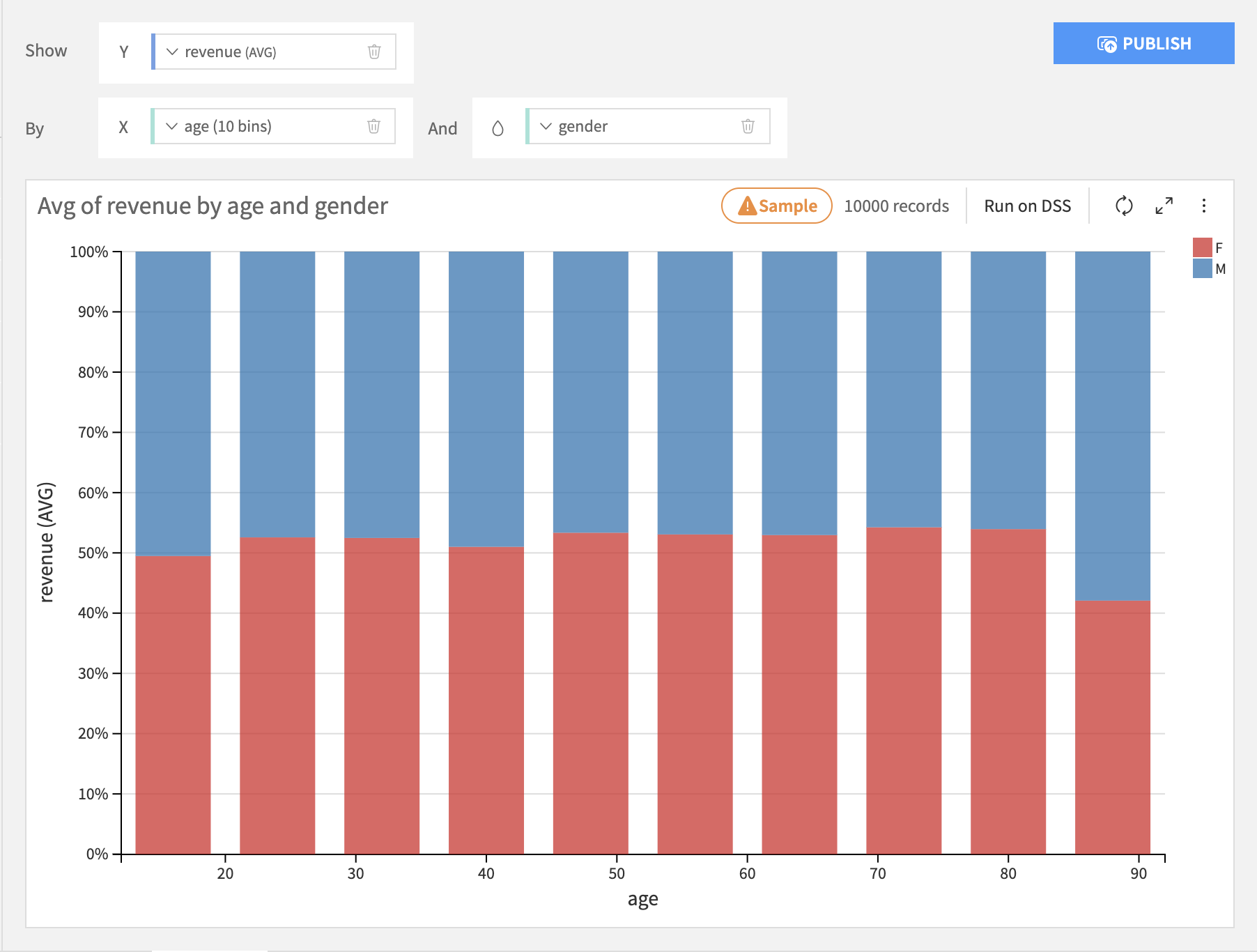• The Bars layout puts the binning column on the Y axis, the summary column on the X axis, and stacks each subgroup within each bin.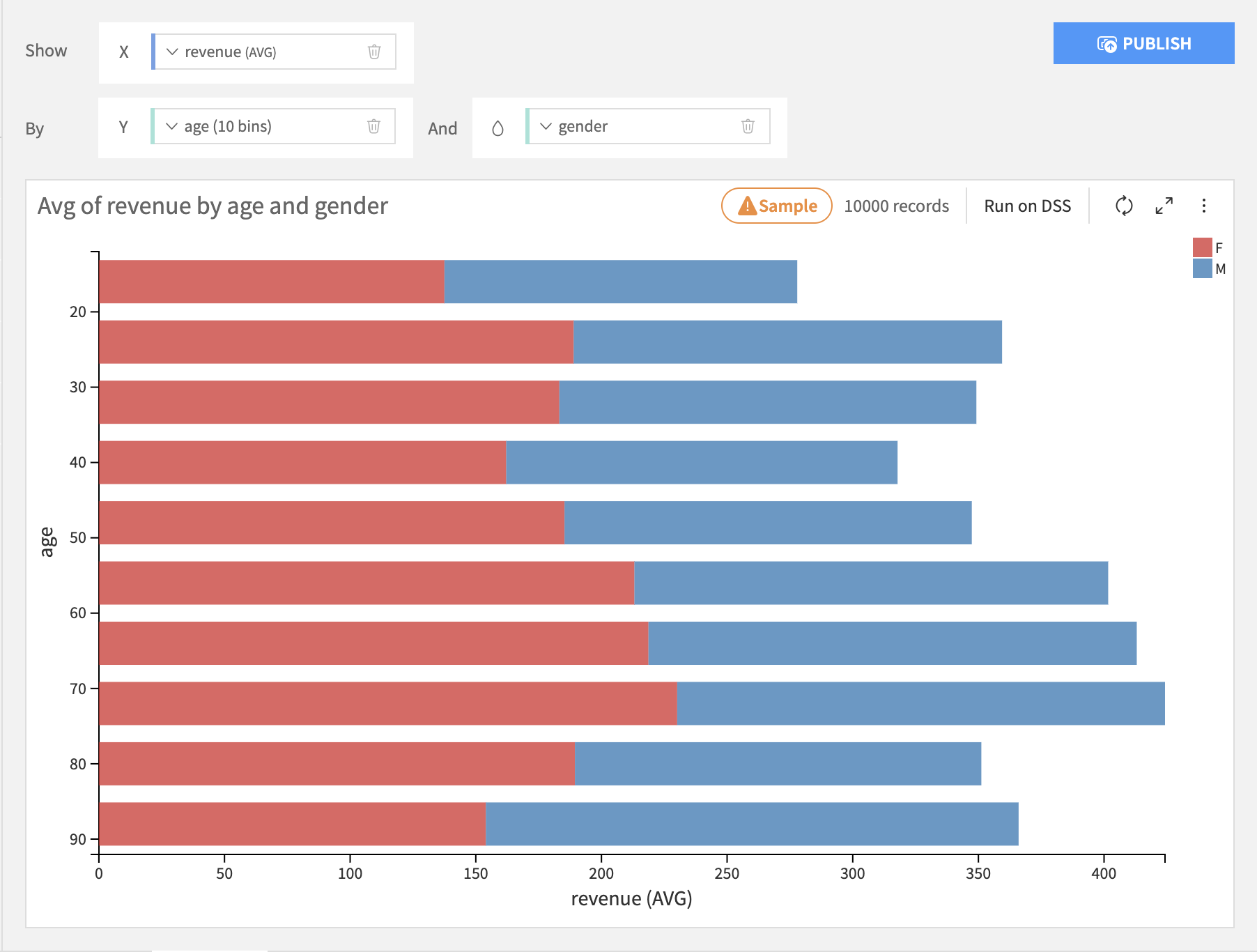• The Bars 100% layout is just like the Bars layout, but the length of each bar is normalized to 100%.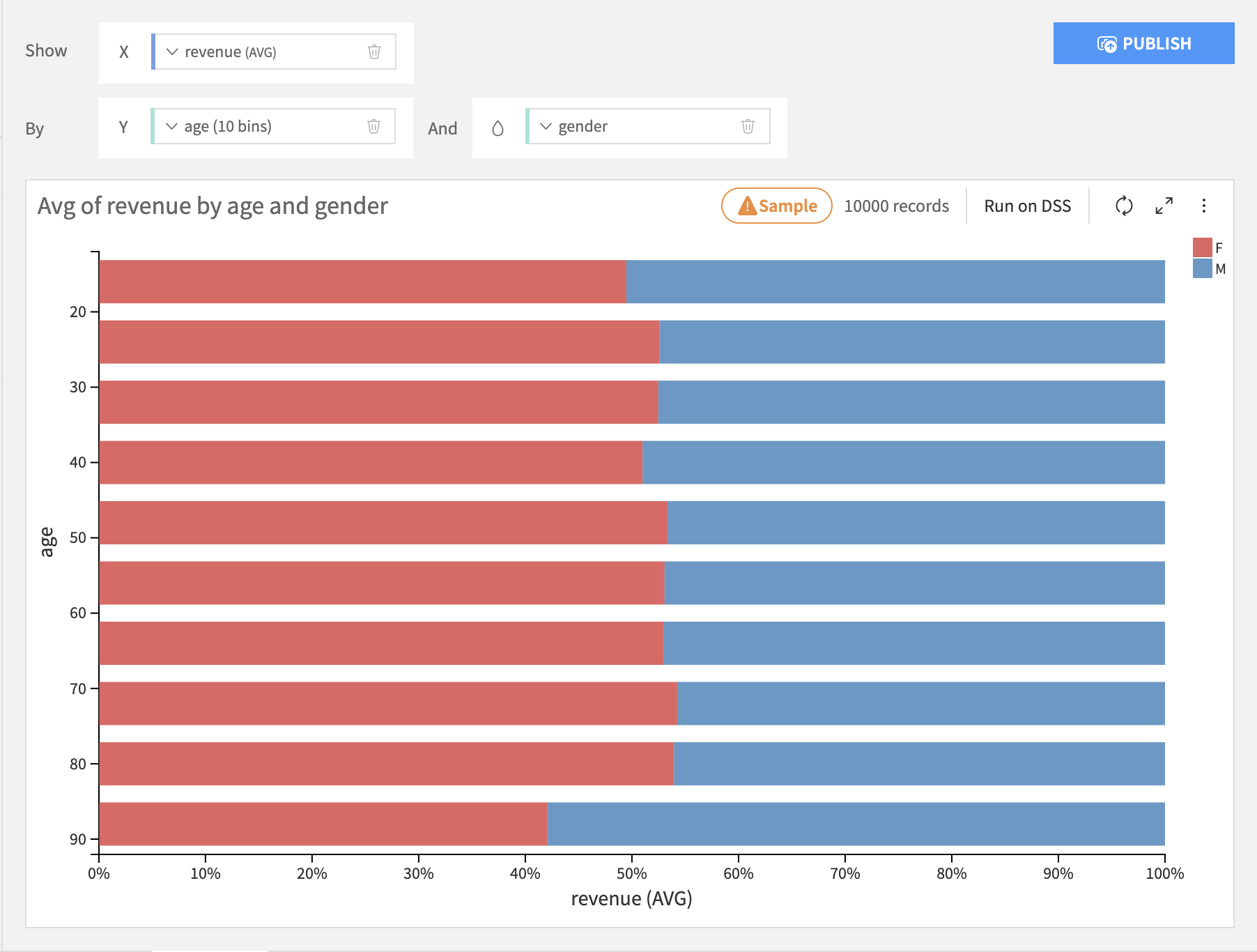### Lines & Curves¶

Lines & curves charts are generally most useful for comparing multiple subgroups or summary columns.

• The Lines layout puts the binning column on the X axis, the line height column on the Y axis, and creates separate lines for each subgroup for each bin.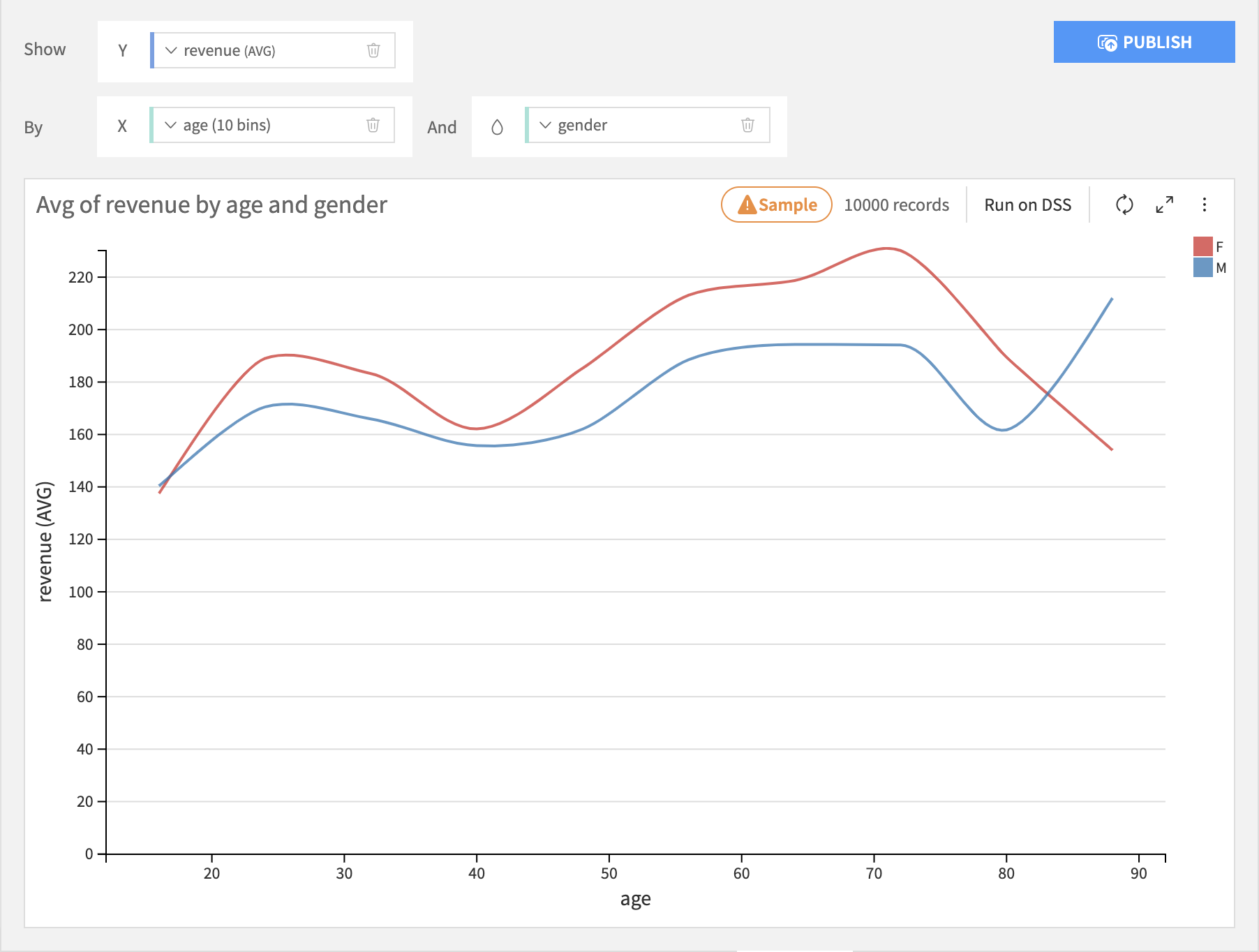• The Mixed layout puts the binning column on the X axis, and for each height column on the Y axis, you can choose whether to display it as a bar or line.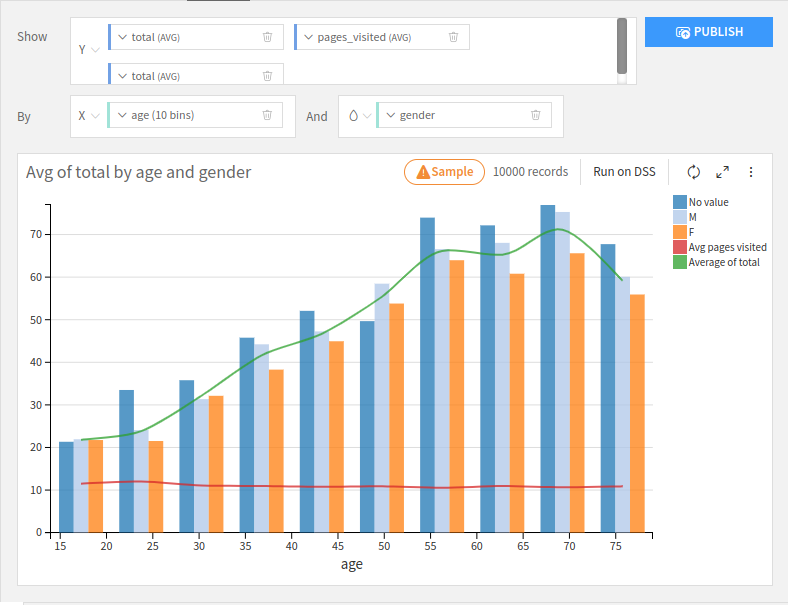• The Stacked Area layout works roughly like a Stacked Bar chart, but it will create a smooth area instead of columns.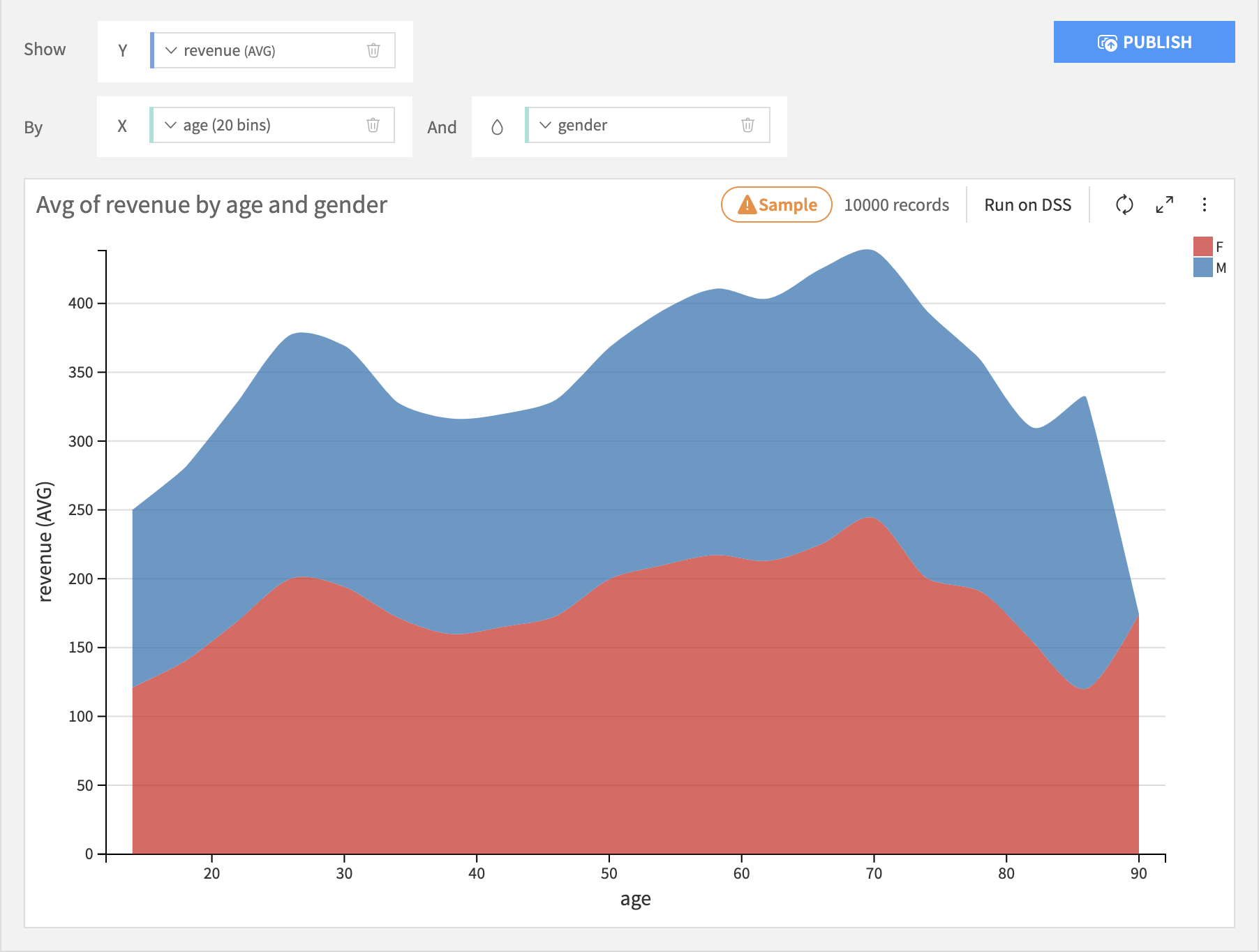• The Stacked Area 100% layout works roughly like a Stacked 100% Bar chart, but it will create a smooth area instead of columns.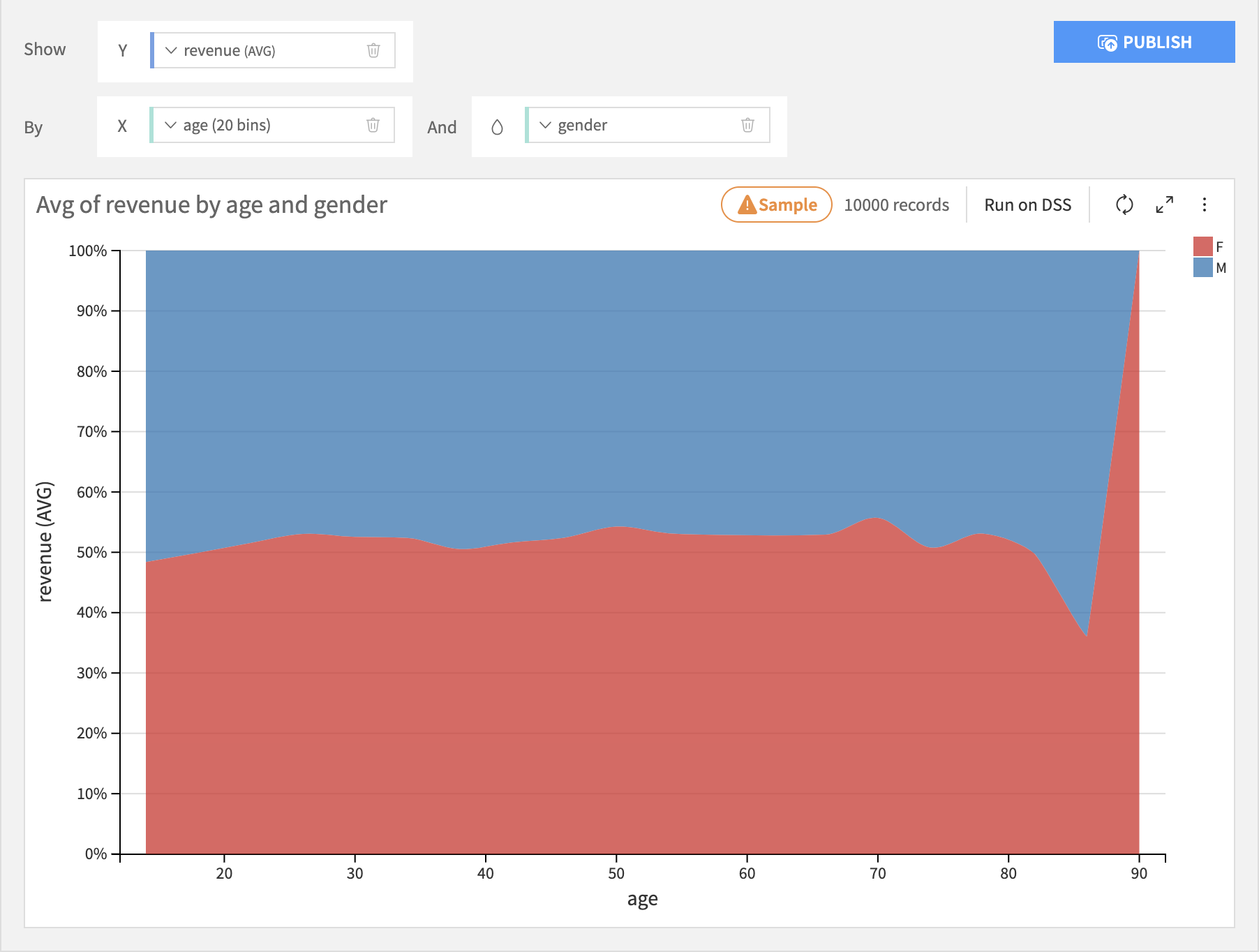### Pie & Donuts¶

Pie & donut charts are generally most appropriate when there is no inherent ordering of the bins.

• Pie charts are like bar charts, but each bin is represented by a wedge in a circle, like a slice of pie. The size of each wedge is proportional to the sum total of all wedges. There is no subgrouping of wedges.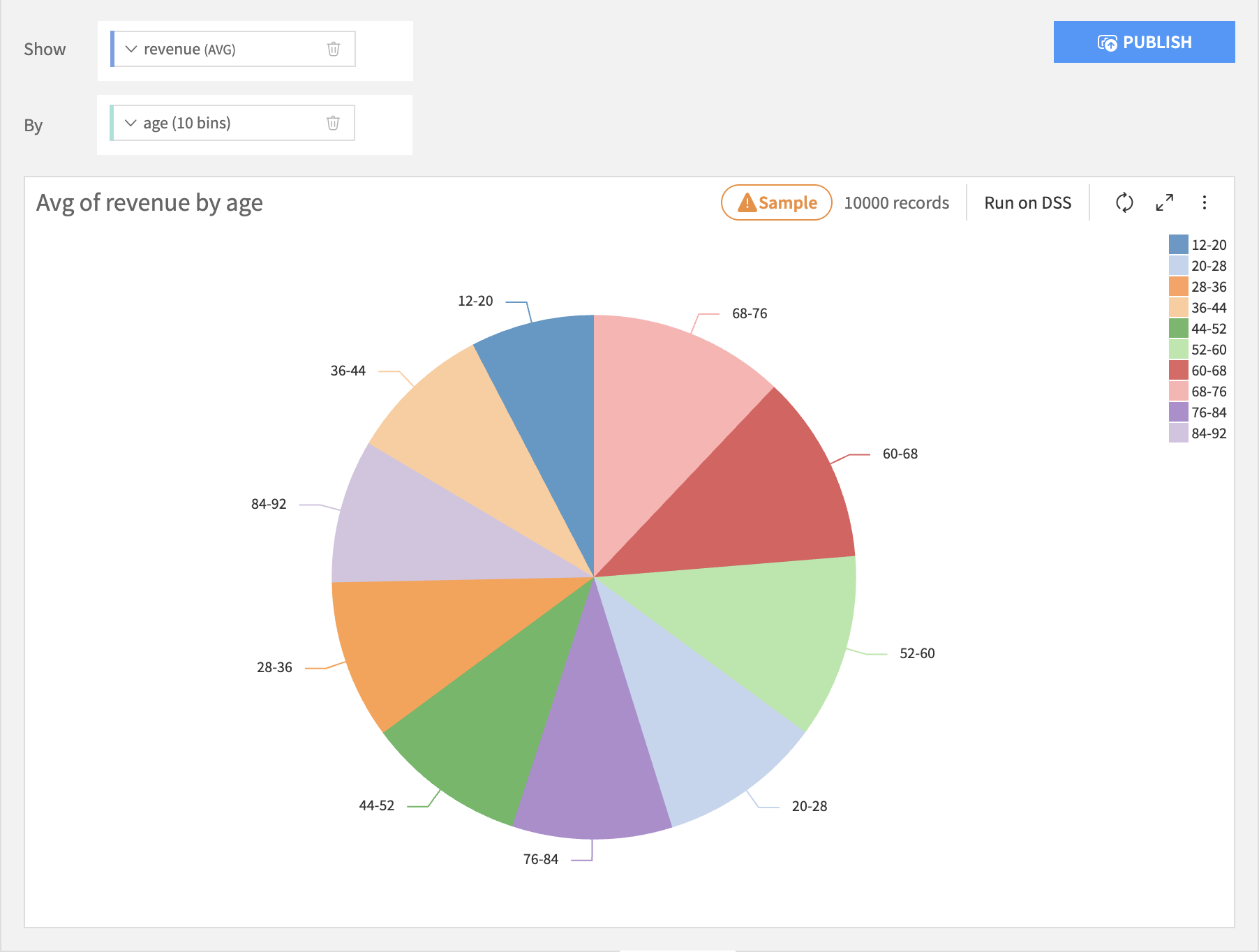• Donut charts are like pie charts, but with the middle removed.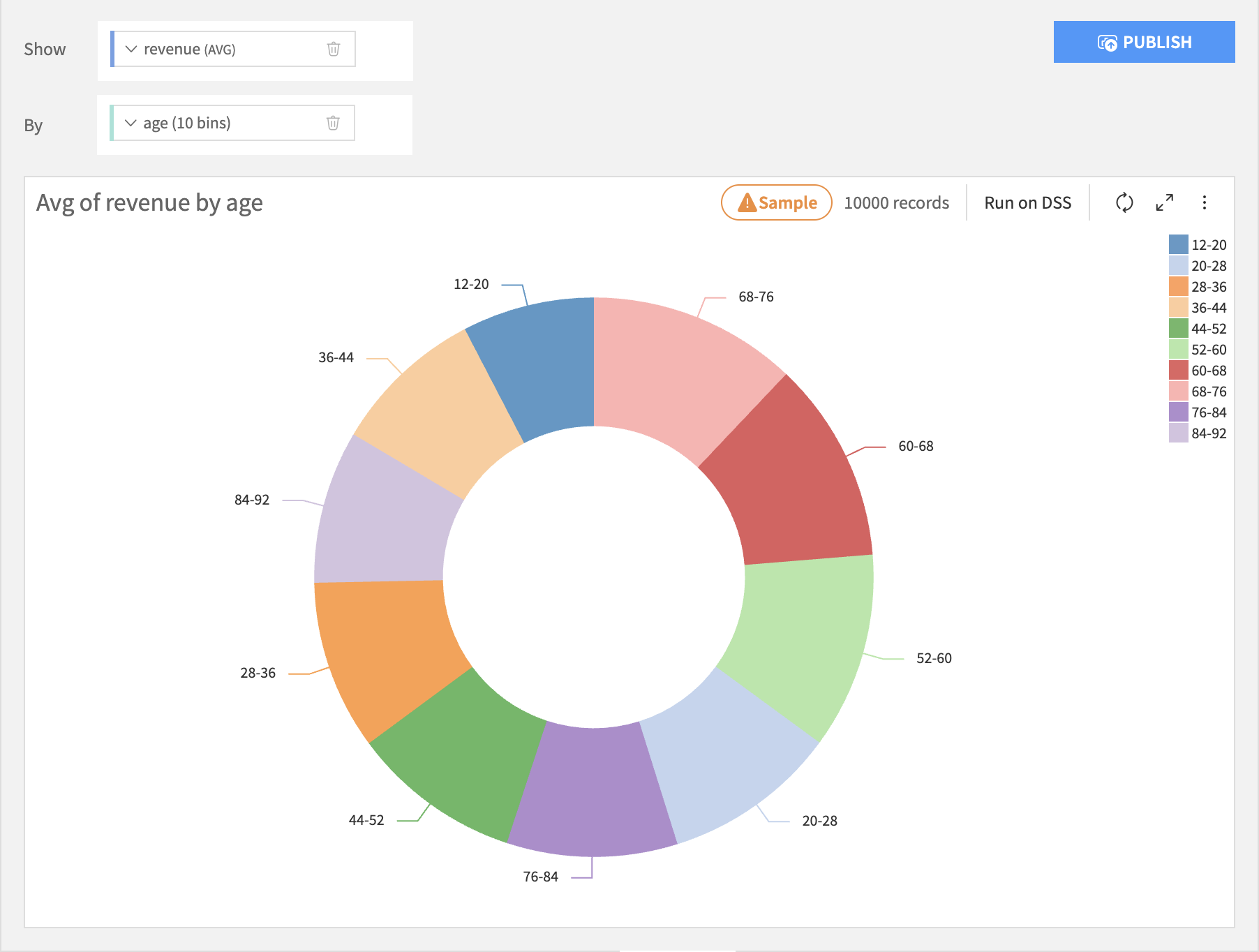## Column Processing Options¶

### Binning Column¶

Set the binning rules by clicking on the name of the binning column.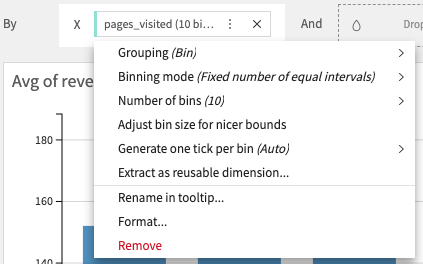### Summary Column¶

Set the aggregation rules by clicking on the name of the summary column.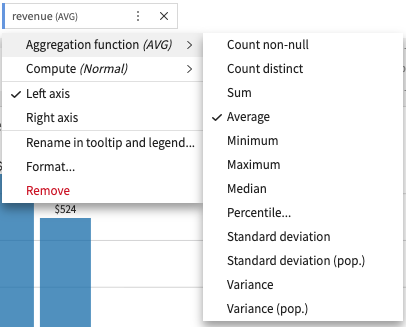### Grouping Column¶

Set the subgroup sorting rules by clicking on the name of the grouping column.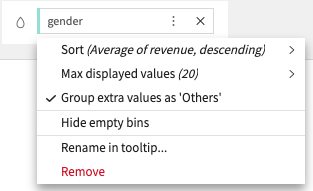Note

It generally does not make sense to use the “AVERAGE” aggregation when creating subgroups of of bars. Only aggregations that “naturally stack” should be used: SUM and COUNT.